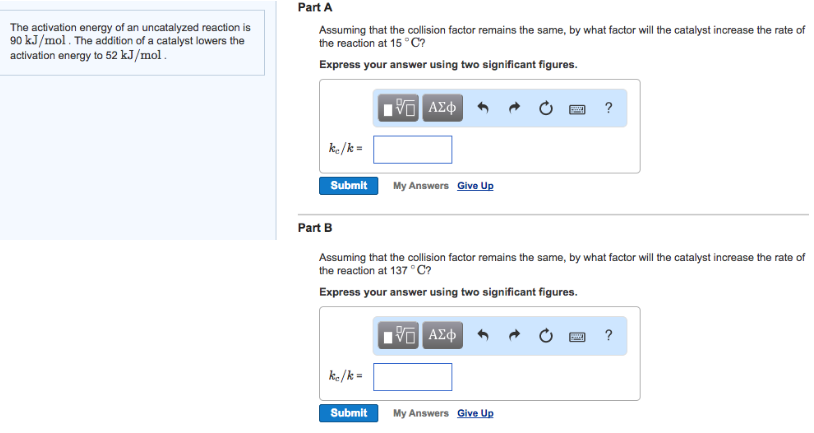# Problem: The activation energy of an uncatalyzed reaction is 90 kJ/mol. The addition of a catalyst lowers the activation energy to 52 kJ/mol. Assuming that the collision factor remains the same, by what factor will the catalyst increase the rate of the reaction at 15°C? Express your answer using two significant figures.Assuming that the collision factor remains the same, by what factor will the catalyst increase the rate of the reaction at 137°C?Express your answer using two significant figures.

###### FREE Expert Solution
97% (239 ratings)###### Problem Details

The activation energy of an uncatalyzed reaction is 90 kJ/mol. The addition of a catalyst lowers the activation energy to 52 kJ/mol.

Assuming that the collision factor remains the same, by what factor will the catalyst increase the rate of the reaction at 15°C?

Assuming that the collision factor remains the same, by what factor will the catalyst increase the rate of the reaction at 137°C?